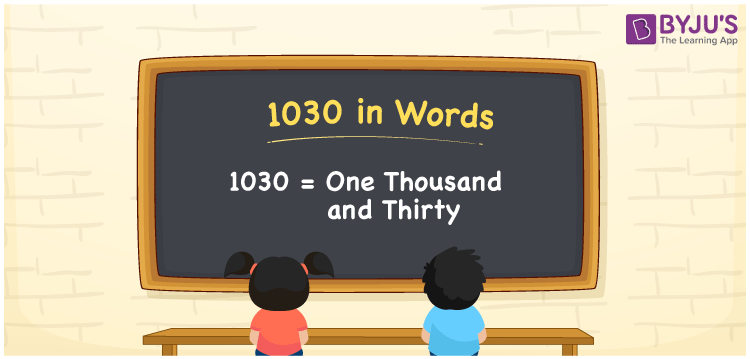# 1030 in Words

One Thousand and Thirty is represented by the number 1030. We can consider 1030 to be a cardinal number because it is used to count things and express values. The place value system can be used to convert the number 1030 to words. If the cost of a book is Rs. 1030, it can be written as “The cost of a book is Rs. One Thousand and Thirty”. Let us look at how to write 1030 in words as well as the technique employed in this article.

 1030 in Words: One Thousand and Thirty. One Thousand and Thirty in Numerical Form: 1030.

## 1030 in English Words## How to Write 1030 in Words?

The number 1030 is written in words using the number’s place value. The place value table for the number 1030 is shown below.

 Thousands Hundreds Tens Ones 1 0 3 0

The expanded form of 1030 is as follows:

= 1 × Thousand + 0 × Hundred + 3 × Ten + 0 × One

= 1 × 1000 + 3 × 10

= 1000 + 30

= 1030

= One thousand and thirty

Hence, 1030 in words is one thousand and thirty.

1030 in words – One thousand and thirty

Is 1030 an odd number? – No

Is 1030 an even number? – Yes

Is 1030 a perfect square number? – No

Is 1030 a perfect cube number? – No

Is 1030 a prime number? – No

Is 1030 a composite number? – Yes

## Frequently Asked Questions on 1030 in Words

Q1

### Write 1030 in words.

1030 in words is one thousand and thirty.

Q2

### Simplify 1000 + 30, and express in words.

Simplifying 1000 + 30, we get 1030. Hence, 1030 in words is one thousand and thirty.

Q3

### Convert one thousand and thirty into numbers.

One thousand and thirty in numbers is 1030.

Test your Knowledge on 1030 in Words